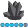## PROC SGPLOT - INSET statement with a BY statement

I am trying to inset some text on some graphs produced in PROC SGPLOT.  My code produces three different plots, and I want to add the corresponding correlations to each plot.  I used the BY statement to get the three different plots, and I used the INSET statement to place the correlations on the plots.  However, I don't know how to specify which correlations go to which plot.  Currently, all three correlations are being placed on all three plots.  Does anyone know how to address this problem?

Here is a snippet of my code:

proc sgplot data=timeondrug;

by drug;

scatter x=priortime y=duration;

reg x=priortime y=duration;

xaxis label="Duration on Prior Study (weeks)";

yaxis label="Duration on Endocyte Study (weeks)";

title 'Endocyte Therapy vs. Prior Therapy';

inset ("EC0225" = "&corr225."

"EC0489" = "&corr489."

"EC145" = "&corr145.") / border title='Correlation';

run;AncaTilea
Pyrite | Level 9

## Re: PROC SGPLOT - INSET statement with a BY statement

Hi.

proc sort data = sashelp.class out = foo;by sex;

data foo;

length text \$20.;

set foo;

by sex;

if first.sex then do;

xPosition = 1;

yPosition = 20;

text = "Corr = 0.15";*replace with your macro variable;

end;

else do;

xPosition = 1;

yPosition = 20;

text = "Corr = 0.25";*replace with your macro variable;

end;

run;

proc sgpanel data=foo noautolegend;

panelby sex;

scatter y=yPosition x=xPosition/ markerchar = text markercharattrs = (weight=bold);*this plots the "values for your correlation at x = 1 and y = 20 coordinates;

scatter y =weight x=age;

reg x=age y=weight;

title 'Some title';

run;

Discussion stats